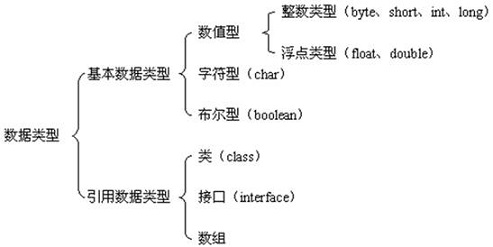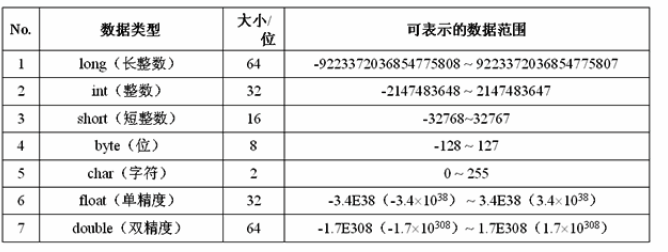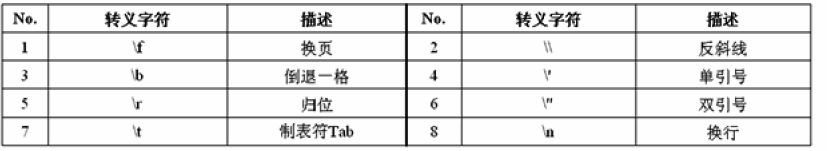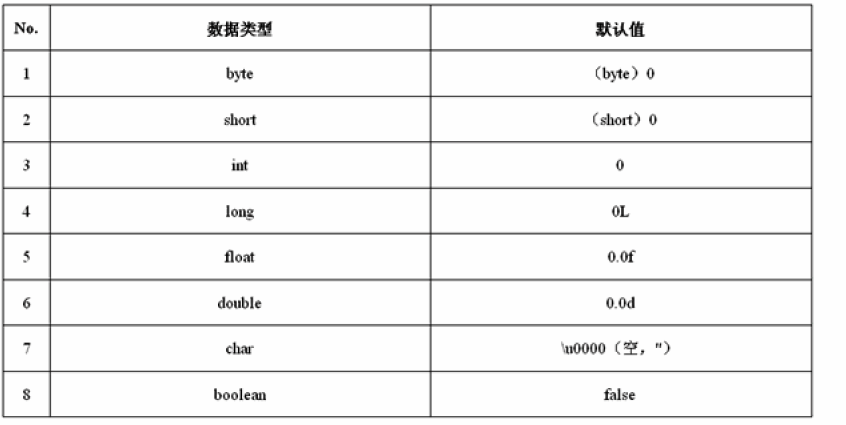#### Java数据类型划分• 基本数据类型：类似于普通的值。
• 引用数据类型：传递的是内存的地址。

#### Java基本数据类型#### 数据的溢出

``````public class T {
public static void main(String[] args) {
int max = Integer.MAX_VALUE;
System.out.println("整型的最大值为："+max); //整型的最大值为：2147483647
}
}
``````

``````public class T {
public static void main(String[] args) {
int max = Integer.MAX_VALUE;
System.out.println("整型的最大值为："+max);       //整型的最大值为：2147483647
System.out.println("整型的最大值+1: "+(max+1));  //整型的最大值+1: -2147483648
System.out.println("整型的最大值+2: "+(max+2));  //整型的最大值+2: -2147483647
}
}
``````

``````public class T {
public static void main(String[] args) {
int max = Integer.MAX_VALUE;
System.out.println("整型的最大值为："+max);       //整型的最大值为：2147483647
System.out.println("整型的最大值+1: "+(max+1));  //整型的最大值+1: -2147483648
System.out.println("整型的最大值+2: "+(max+2));  //整型的最大值+2: -2147483647
System.out.println("整型的最大值+2: "+((long)max+2));  //2147483649
}
}
``````

#### 字符类型

``````public class T {
public static void main(String[] args) {
char ch1 = 'a';     //字符是使用''括起来的数据
char ch2 = 97;      //通过数字定义字符变量
System.out.println("ch1 = "+ch1);
System.out.println("ch2 = "+ch2);
}
}
``````

#### 常用的转义字符#### 浮点数类型与双精度浮点数类型

• 小数(1.1，1.2)的默认类型是double类型
• 整数(1，2，3)的默认类型是int类型

#### 基本数据类型的默认值